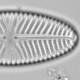## A minimal model for estimating climate sensitivity

One of the classic tells that a fake climate skeptic is trying to squeeze a weak paper into the literature is that they submit it to a journal where it falls outside the usual scope of papers published there. The problem is that the editor is not familiar with the relevant literature nor with the most suitable reviewers and the paper does not get the robust peer review that it might get at a more appropriate journal. Roy Spencer’s paper in Remote Sensing is an exemplar of this ruse. (Someone wrote an article about this and other schemes fake skeptics use for publishing, but I cannot find it now.)

So when I see Craig Loehle’s new paper “A minimal model for estimating climate sensitivity” breathlessly announced by the ever credulous Anthony Watts, my scepticism is raised several notches when I see it is published in Ecological Modelling, rather than a journal with a climate focus.

We should not, of course, condemn this paper just because it is published in an inappropriate journal. There are many more reasons.

Loehle attempts to isolate the anthropogenic signal in the instrumental climate record by removing the “natural multi-decadal cycles”. The anthropogenic trend is then compared with the increase in log(CO2) to calculate transient climate sensitivity. This procedure is described as ” fewest possible assumptions and the least data uncertainty”. What could possibly go wrong?

Natural multidecadal climate variability, due to internal variability such as the Atlantic multidecadal oscillation and variability in external solar forcing, undoubtedly complicates the calculation of climate sensitivity from instrumental data: if it could be removed, calculations of sensitivity would be much easier. Loehle estimates natural multi-decadal variability as the sum of a linear trend and sine waves with periods of 20 and 60 years. This model is fitted to the data pre-1950 and the difference between predictions for this model and the observed climate 1950-present is claimed to represent anthropogenic forcing and noise.

The model of natural variability is devoid of physics, it is simply a curve fitting exercise with a large number of free parameters. Here is the formula

temperature~b0+b1*year+b2*sin(2*pi*(year-b3)/20)+b4*sin(2*pi*(year-b5)/60)

The source of the 20 and 60 year periods is not specified in the paper. Given that there is 100 years of data before 1950, when the anthropogenic is assumed to become detectable, it would obviously be madness to try to estimate the periodicity of 60 years from these data. These periods come from Loehle and Scafetta (2011) who estimate them from the Sun’s movement about the barycentre. Yes this is completely crazy. Even the sun does not respond on a 60 or 20 year cycle (the Gleißberg cycle is ~87 years and the Hale cycle is ~22 years), so there is absolutely no reason to suppose that interval variability in the Earth’s climate should respond to the location of the Sun relative to the barycentre.

The silliness does not end there. Loehle assumes that his predictions of natural climate variability are without error. Even if the model of natural variability was sensible, it would have uncertainties which should be propagated into the estimate of climate sensitivity. By ignoring these uncertainties Loehle artificially deflates the uncertainty on the climate sensitivity

Ignoring for the moment that the model is ridiculous, how much has the uncertainty been underestimated?

Loehle uses, but does not cite, the HadCrut3 dataset. I’m going to use the HadCrut4 dataset, any differences will be minor.

```had<-read.table("http://www.metoffice.gov.uk/hadobs/hadcrut4/data/current/time_series/HadCRUT.4.2.0.0.annual_ns_avg.txt")[,1:2]

```

Now lets fit the model of natural variability for the pre-1950 data using non-linear least squares regression.

```library(nlme)
mod<-nls(temperature~b0+b1*year+b2*sin(2*3.141593*(year-b3)/20)+b4*sin(2*3.141593*(year-b5)/60), data=had, subset=year<=1950, start=c(b0=0, b1=0, b2=1, b3=0, b4=1, b5=0))#using 3.14 rather than pi to avoid problems later

```

Ideally we would use `predict` with the argument `interval="confidence"`, but that argument is not yet implemented in R (there is some uncertainty about how best to do it). Instead I can use a Monte Carlo procedure developed by A. N. Spiess

```predc<-predictNLS(mod, newdata=cbind(had, pi=pi))
```HadCrut4 global temperatures (black) with Loehle’s model of natural climate variability fitted in the blue portion of the solid curve, predicted in the red. The dashed red lines show the 95% MC confidence interval.

The uncertainty, which Loehle ignores, is broad. The uncertainty would be broader still if we relax the assumption that multidecadal natural climate variability consists of just a linear trend and sine waves with a 20 and 60 year period. This will have a large impact on the estimate of climate sensitivity.

Loehle minimal model might have the fewest possible assumptions, but they are not the most sensible.

Loehle, C. 2014. A minimal model for estimating climate sensitivity. Ecological Modelling, 276, 80–841.Sou says: